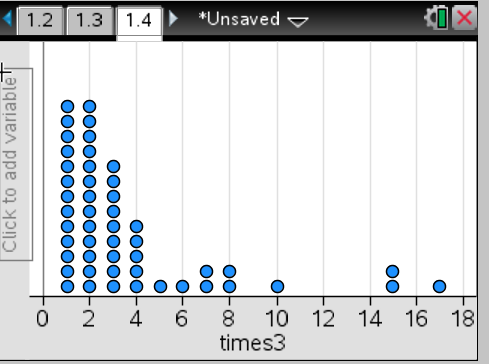# Statistics and Probability / Intro to Data

Activity 1 of 24
The lesson helps students develop a sense of the distribution of data, the number of times each possible outcome occurs.

## Planning and Resources

Objectives
Students should understand the idea of a distribution of a set of data.   They can identify clusters, peaks, and gaps, and recognize common shapes in graphical representations of data (skewed, symmetric, uniform, mound shaped).

Vocabulary
distribution
symmetric
mound shaped
uniform
skewed
range
outlier

Standard:

## Lesson Snapshot

#### Understanding

Students are introduced to the notion of a statistical question, a question that anticipates variability in the response. This helps to set the stage for this series of lessons.

### What to look for

As students compare data, be sure they use the appropriate vocabulary to describe the distributions.

### Sample Assessment

The times it took participants to complete a marathon are shown in the graph below. What is the range of the times?a. 1 to 17 hours
b. 16 hours
c. 17 hours
d. 1 to 2 hours